Скачать презентацию Erasing correlations destroying entanglement and other new challenges

a2a6f3625852d65d38c440c0d72ffbcd.ppt

• Количество слайдов: 18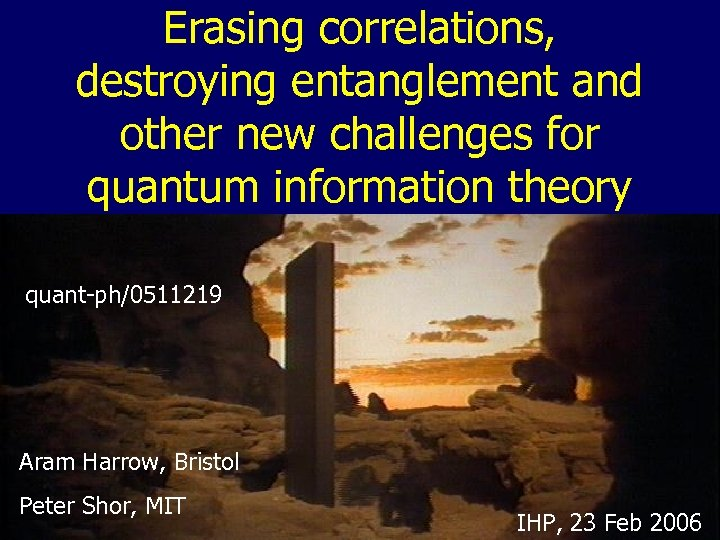Erasing correlations, destroying entanglement and other new challenges for quantum information theory quant-ph/0511219 Aram Harrow, Bristol Peter Shor, MIT IHP, 23 Feb 2006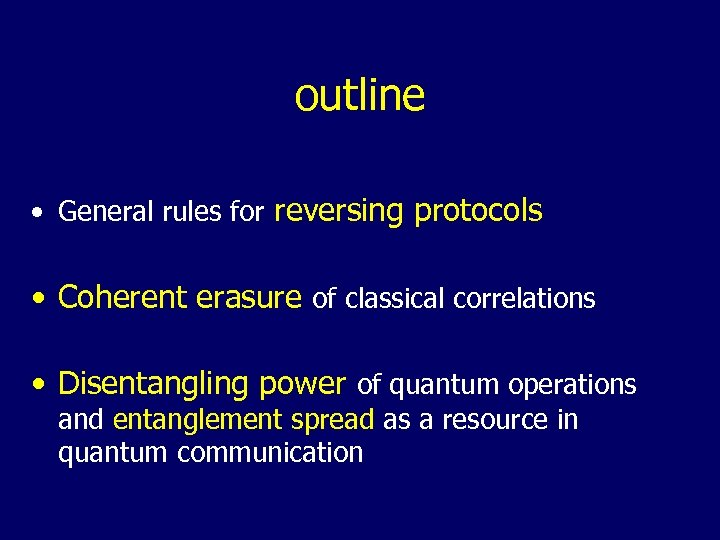outline • General rules for reversing protocols • Coherent erasure of classical correlations • Disentangling power of quantum operations and entanglement spread as a resource in quantum communication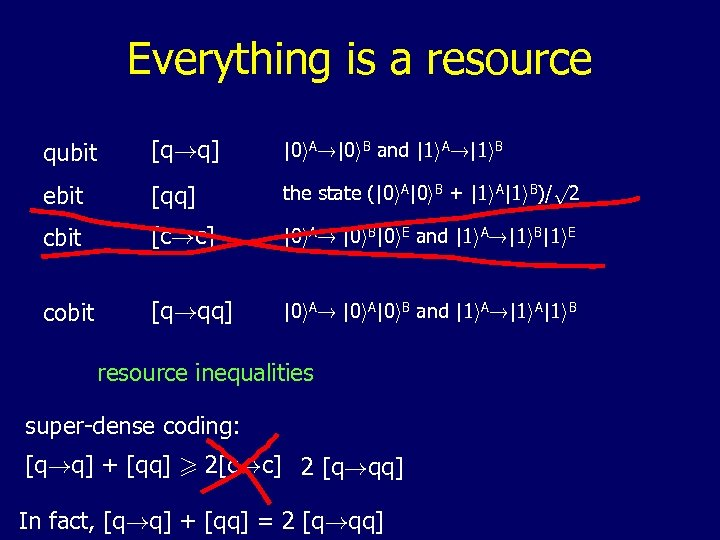Everything is a resource qubit [q!q] |0 i. A!|0 i. B and |1 i. A!|1 i. B ebit [qq] the state (|0 i. A|0 i. B + |1 i. A|1 i. B)/p 2 cbit [c!c] |0 i. A! |0 i. B|0 i. E and |1 i. A!|1 i. B|1 i. E cobit [q!qq] |0 i. A! |0 i. A|0 i. B and |1 i. A!|1 i. A|1 i. B resource inequalities super-dense coding: [q!q] + [qq] > 2[c!c] 2 [q!qq] In fact, [q!q] + [qq] = 2 [q!qq]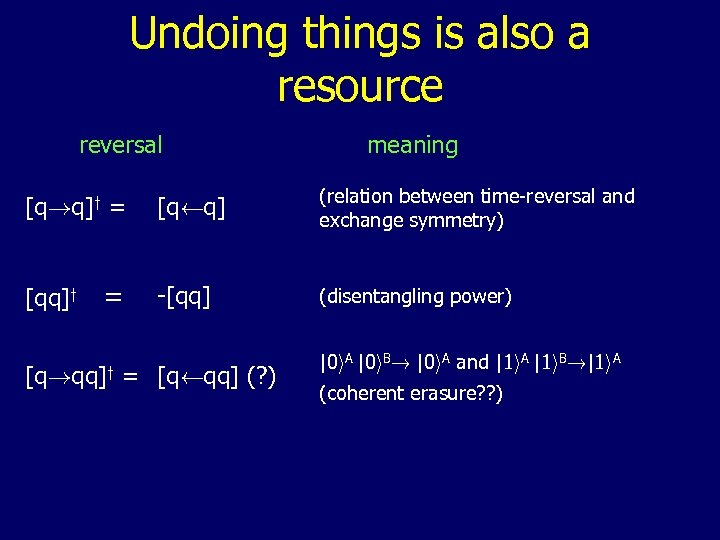Undoing things is also a resource reversal [q!q]y = [qq]y = meaning [qÃq] (relation between time-reversal and exchange symmetry) -[qq] (disentangling power) [q!qq]y = [qÃqq] (? ) |0 i. A |0 i. B! |0 i. A and |1 i. A |1 i. B!|1 i. A (coherent erasure? ? )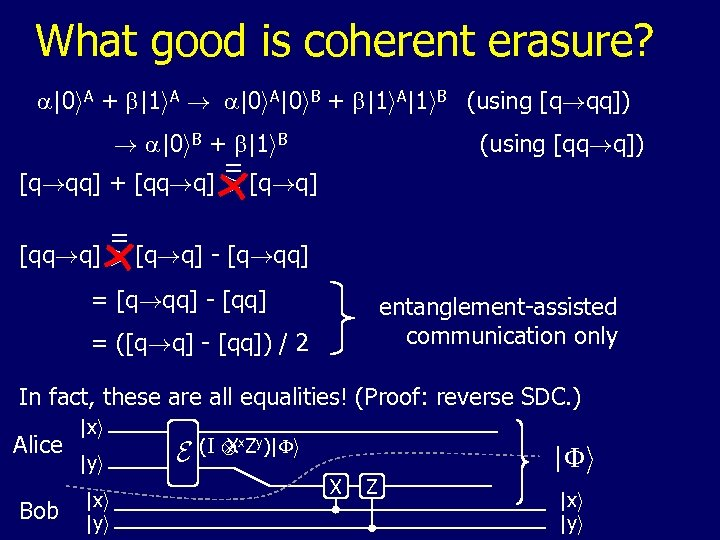What good is coherent erasure? a|0 i. A + b|1 i. A ! a|0 i. A|0 i. B + b|1 i. A|1 i. B (using [q!qq]) ! a|0 i. B + b|1 i. B (using [qq!q]) = [q!qq] + [qq!q] > [q!q] = [qq!q] > [q!q] - [q!qq] = [q!qq] - [qq] entanglement-assisted communication only = ([q!q] - [qq]) / 2 In fact, these are all equalities! (Proof: reverse SDC. ) Alice Bob |xi |yi E (I x. Zy)|Fi X Z |xi |yi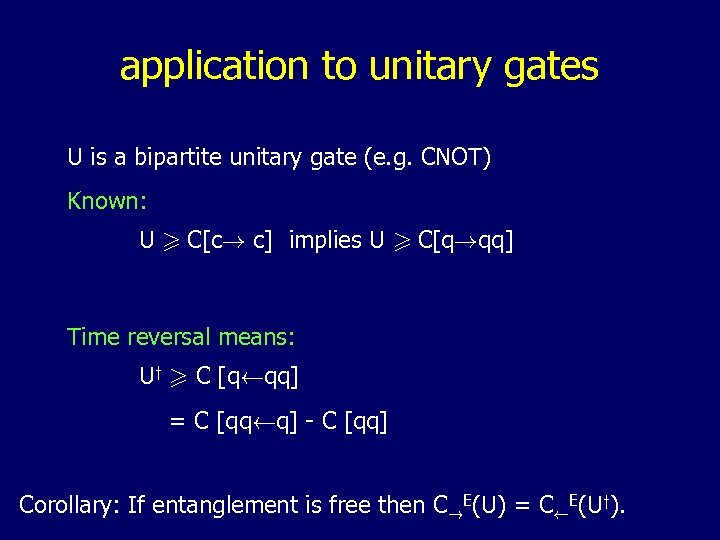application to unitary gates U is a bipartite unitary gate (e. g. CNOT) Known: U > C[c! c] implies U > C[q!qq] Time reversal means: Uy > C [qÃqq] = C [qqÃq] - C [qq] Corollary: If entanglement is free then C!E(U) = CÃE(Uy).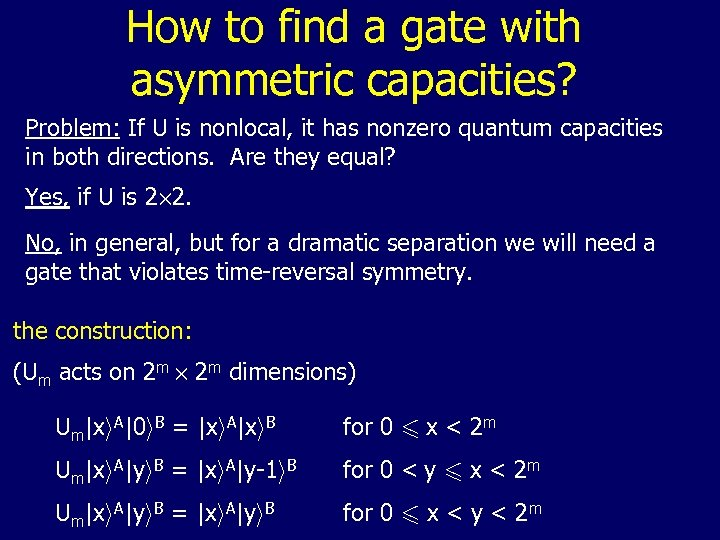How to find a gate with asymmetric capacities? Problem: If U is nonlocal, it has nonzero quantum capacities in both directions. Are they equal? Yes, if U is 2£ 2. No, in general, but for a dramatic separation we will need a gate that violates time-reversal symmetry. the construction: (Um acts on 2 m £ 2 m dimensions) Um|xi. A|0 i. B = |xi. A|xi. B for 0 6 x < 2 m Um|xi. A|yi. B = |xi. A|y-1 i. B for 0 < y 6 x < 2 m Um|xi. A|yi. B = |xi. A|yi. B for 0 6 x < y < 2 m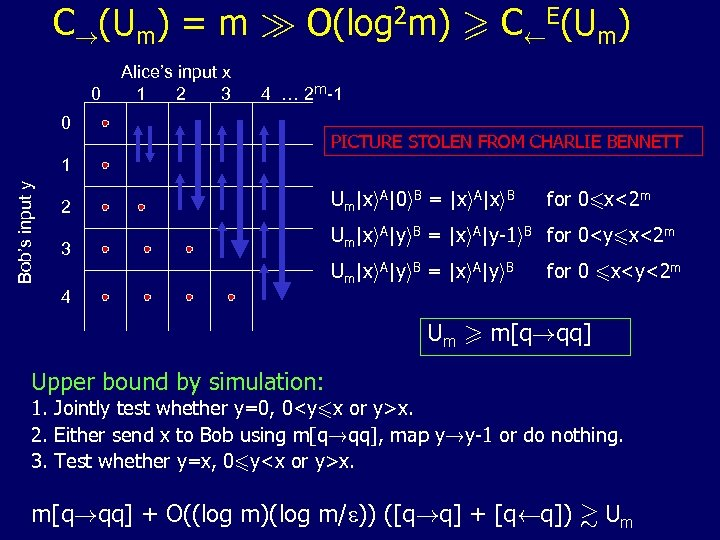C!(Um) = m À O(log 2 m) > CÃE(Um) 0 Alice’s input x 1 2 3 4 … 2 m-1 0 PICTURE STOLEN FROM CHARLIE BENNETT Bob’s input y 1 2 3 4 Um|xi. A|0 i. B = |xi. A|xi. B for 06 x<2 m Um|xi. A|yi. B = |xi. A|y-1 i. B for 0 m[q!qq] Upper bound by simulation: 1. Jointly test whether y=0, 0x. 2. Either send x to Bob using m[q!qq], map y!y-1 or do nothing. 3. Test whether y=x, 06 yx. m[q!qq] + O((log m)(log m/e)) ([q!q] + [qÃq]) & Um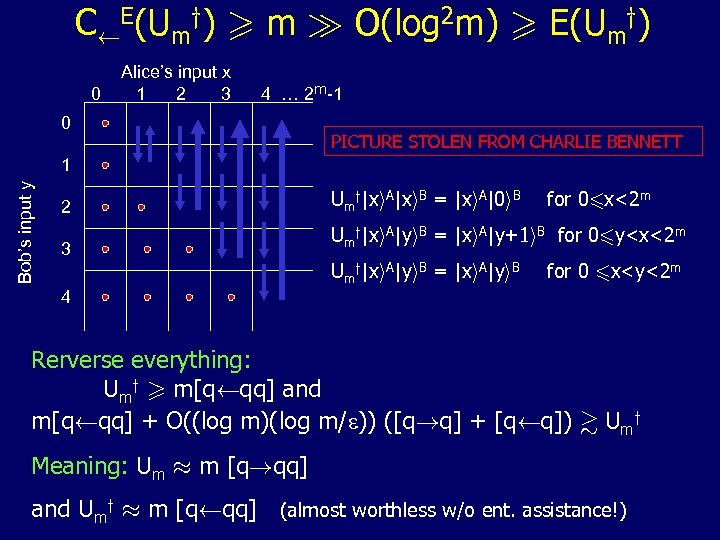CÃE(Umy) > m À O(log 2 m) > E(Umy) 0 Alice’s input x 1 2 3 4 … 2 m-1 0 PICTURE STOLEN FROM CHARLIE BENNETT Bob’s input y 1 2 3 4 Umy|xi. A|xi. B = |xi. A|0 i. B for 06 x<2 m Umy|xi. A|yi. B = |xi. A|y+1 i. B for 06 y m[qÃqq] and m[qÃqq] + O((log m)(log m/e)) ([q!q] + [qÃq]) & Umy Meaning: Um ¼ m [q!qq] and Umy ¼ m [qÃqq] (almost worthless w/o ent. assistance!)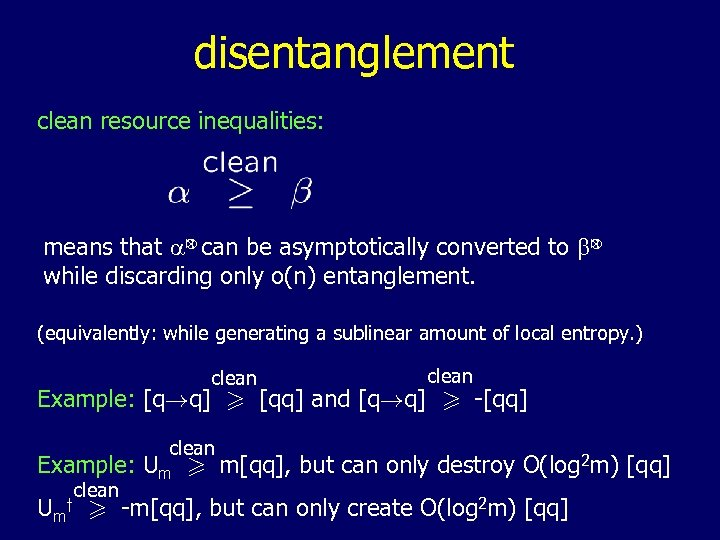disentanglement clean resource inequalities: n n means that a can be asymptotically converted to b while discarding only o(n) entanglement. (equivalently: while generating a sublinear amount of local entropy. ) clean Example: [q!q] > [qq] and [q!q] > -[qq] clean Example: Um > m[qq], but can only destroy O(log 2 m) [qq] clean Um > -m[qq], but can only create O(log 2 m) [qq] y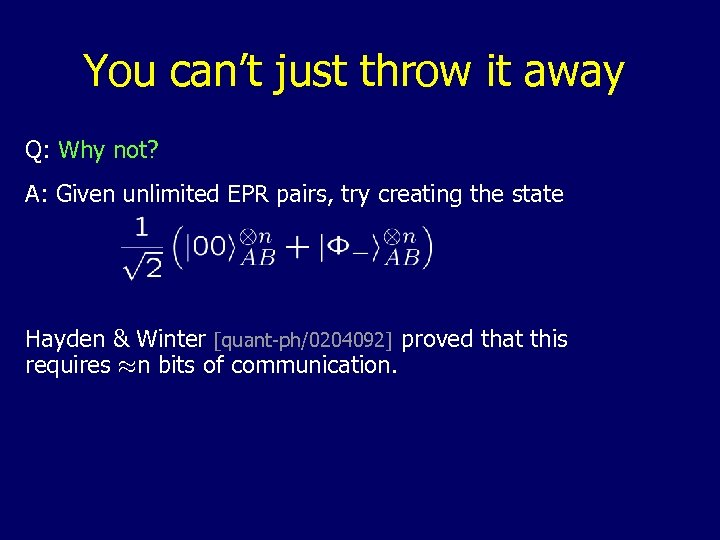You can’t just throw it away Q: Why not? A: Given unlimited EPR pairs, try creating the state Hayden & Winter [quant-ph/0204092] proved that this requires ¼n bits of communication.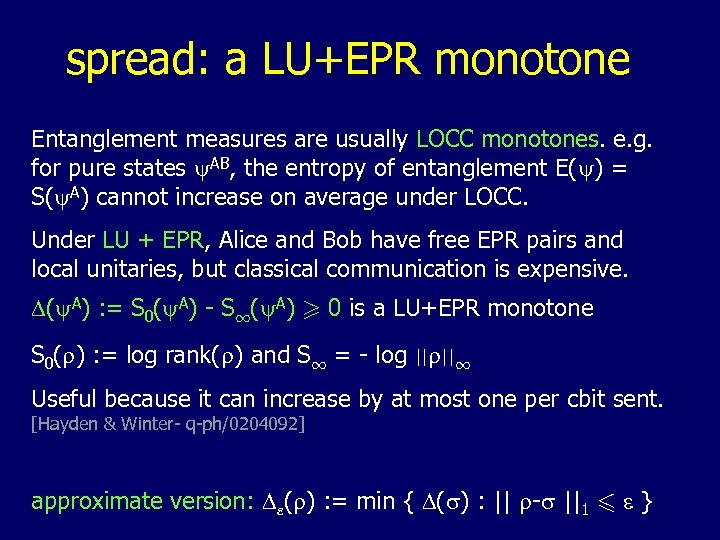spread: a LU+EPR monotone Entanglement measures are usually LOCC monotones. e. g. for pure states y. AB, the entropy of entanglement E(y) = S(y. A) cannot increase on average under LOCC. Under LU + EPR, Alice and Bob have free EPR pairs and local unitaries, but classical communication is expensive. D(y. A) : = S 0(y. A) - S 1(y. A) > 0 is a LU+EPR monotone S 0(r) : = log rank(r) and S 1 = - log ||r||1 Useful because it can increase by at most one per cbit sent. [Hayden & Winter- q-ph/0204092] approximate version: De(r) : = min { D(s) : || r-s ||1 6 e }spread: another resource you didn’t know you needed 1. Entanglement dilution: |yi. AB is partially entangled. E = S(y. A). n n goal: |Fi E+o(n)!|yi 1 n even the weaker |Fi !|yi requires W(n½) cbits (in either direction). why? concentration requires no communication: n n |yi ! |Fi E+d with probability p(d), where p(d)¼Gaussian with mean 0 and std. dev O(n½), n n meaning |yi @LU åd pp(d)|di. A|di. B |Fi E+d n n Thus D((y. A) ) ~ n½ and creating |yi means creating O(n½) entanglement spread, which requires communication. (possible [Lo&Popescu, q-ph/9902045], necessary [Hayden&Winter, q-ph/0204092; Harrow&Lo, q-ph/0204096])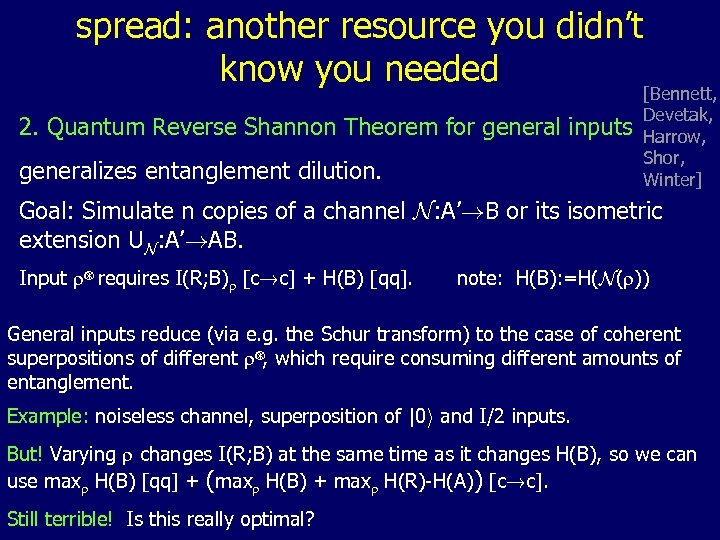spread: another resource you didn’t know you needed 2. Quantum Reverse Shannon Theorem for general inputs generalizes entanglement dilution. [Bennett, Devetak, Harrow, Shor, Winter] Goal: Simulate n copies of a channel N: A’!B or its isometric extension UN: A’!AB. n Input r requires I(R; B)r [c!c] + H(B) [qq]. note: H(B): =H(N(r)) General inputs reduce (via e. g. the Schur transform) to the case of coherent n superpositions of different r , which require consuming different amounts of entanglement. Example: noiseless channel, superposition of |0 i and I/2 inputs. But! Varying r changes I(R; B) at the same time as it changes H(B), so we can use maxr H(B) [qq] + (maxr H(B) + maxr H(R)-H(A)) [c!c]. Still terrible! Is this really optimal?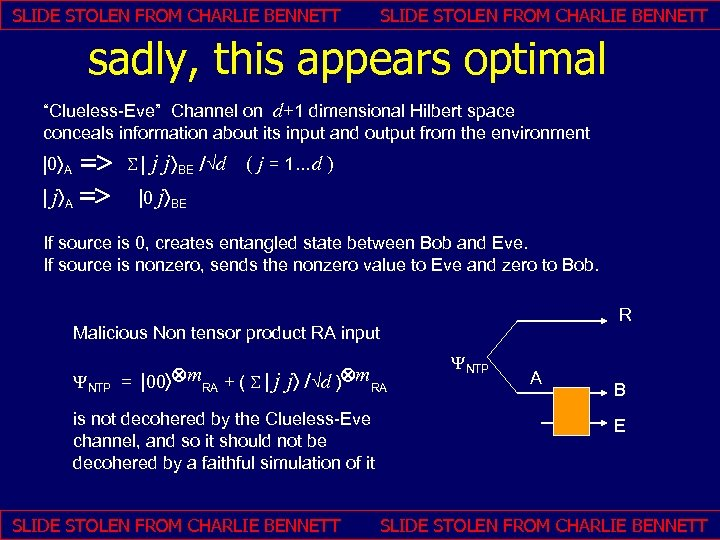SLIDE STOLEN FROM CHARLIE BENNETT sadly, this appears optimal “Clueless-Eve” Channel on d+1 dimensional Hilbert space conceals information about its input and output from the environment => | jñA => | 0 ñA j jñBE /Öd ( j = 1…d ) |0 jñBE S| If source is 0, creates entangled state between Bob and Eve. If source is nonzero, sends the nonzero value to Eve and zero to Bob. R Malicious Non tensor product RA input YNTP = |00ñÄm. RA + ( S | j jñ /Öd )Äm. RA is not decohered by the Clueless-Eve channel, and so it should not be decohered by a faithful simulation of it SLIDE STOLEN FROM CHARLIE BENNETT YNTP A B E SLIDE STOLEN FROM CHARLIE BENNETT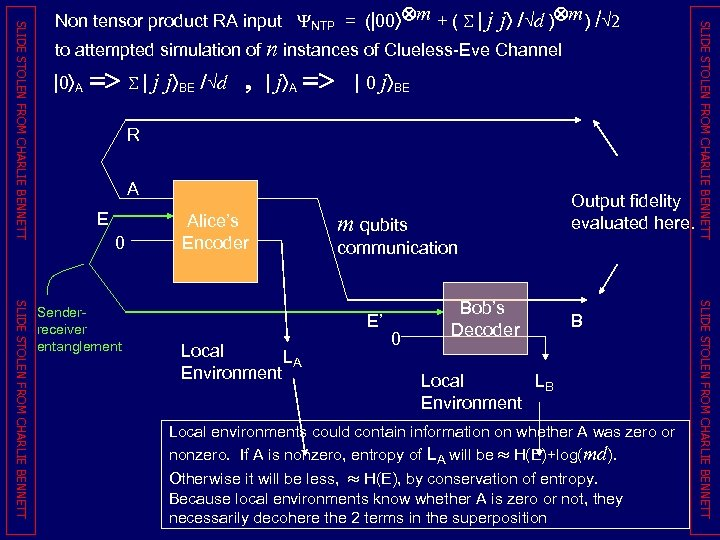to attempted simulation of n instances of Clueless-Eve Channel | 0 ñA => S | j jñBE /Öd , | jñA => | 0 jñBE R A E 0 m qubits communication E’ Local LA Environment 0 Bob’s Decoder B Local LB Environment Local environments could contain information on whether A was zero or nonzero. If A is nonzero, entropy of LA will be » H(E)+log(md). Otherwise it will be less, » H(E), by conservation of entropy. Because local environments know whether A is zero or not, they necessarily decohere the 2 terms in the superposition SLIDE STOLEN FROM CHARLIE BENNETT Senderreceiver entanglement Alice’s Encoder Output fidelity evaluated here. SLIDE STOLEN FROM CHARLIE BENNETT Non tensor product RA input YNTP = (|00ñÄm + ( S | j jñ /Öd )Äm) /Ö 2Spread: where to buy it 1. Classical communication in either direction: n x n |xi. A|xi. B|Fi. AB ! |xi. A|xi. B|Fi |00 i -x uses POVM with elements 2. embezzle it! [q-ph/0205100, Hayden-van Dam] spread n with error e using a size S=n/e embezzling state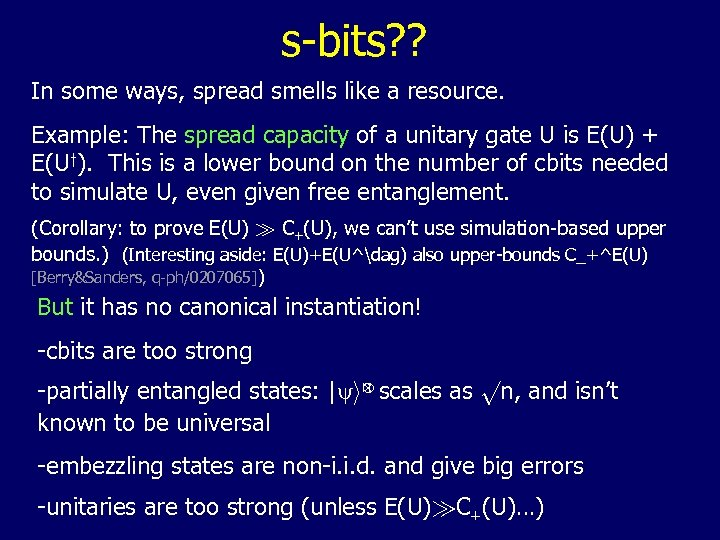s-bits? ? In some ways, spread smells like a resource. Example: The spread capacity of a unitary gate U is E(U) + E(Uy). This is a lower bound on the number of cbits needed to simulate U, even given free entanglement. (Corollary: to prove E(U) À C+(U), we can’t use simulation-based upper bounds. ) (Interesting aside: E(U)+E(U^dag) also upper-bounds C_+^E(U) [Berry&Sanders, q-ph/0207065]) But it has no canonical instantiation! -cbits are too strong n -partially entangled states: |yi scales as pn, and isn’t known to be universal -embezzling states are non-i. i. d. and give big errors -unitaries are too strong (unless E(U)ÀC+(U)…)